# Length + analytic geometry - math problems

#### Number of problems found: 29

• Segment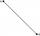Calculate the segment AB's length if the coordinates of the end vertices are A[10, -4] and B[5, 5].
• Distance problemA=(x, x) B=(1,4) Distance AB=√5, find x;
• Calculate 8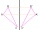Calculate the coordinates of point B axially symmetrical with point A[-1, -3] along a straight line p : x + y - 2 = 0.
• Distance problem 2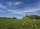A=(x,2x) B=(2x,1) Distance AB=√2, find value of x
• Distance between 2 pointsFind the distance between the points (7, -9), (-1, -9)
• Square side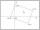Calculate length of side square ABCD with vertex A[0, 0] if diagonal BD lies on line p: -4x -5 =0.
• Right triangle - leg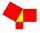Calculate to the nearest tenth cm length of leg in right-angled triangle with hypotenuse length 9 cm and 7 cm long leg.
• Triangle IRTIn isosceles right triangle ABC with right angle at vertex C is coordinates: A (-1, 2); C (-5, -2) Calculate the length of segment AB.
• Vertex pointsGiven the following points of a triangle: P(-12,6), Q(4,0), R(-8,-6). Graph the triangle. Find the triangle area.
• Four sides of trapezoidTrapezoid is given by length of four sides: 40.5 42.5 52.8 35.0. Calculate its area.
• Three pointsThree points A (-3;-5) B (9;-10) and C (2;k) . AB=AC What is value of k?
• Calculate 6Calculate the distance of a point A[0, 2] from a line passing through points B[9, 5] and C[1, -1].
• Parametric formCalculate the distance of point A [2,1] from the line p: X = -1 + 3 t Y = 5-4 t Line p has a parametric form of the line equation. ..
• Coordinate axes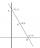Determine the area of the triangle given by line -7x+7y+63=0 and coordinate axes x and y.
• Perpendicular projectionDetermine the distance of a point B[1, -3] from the perpendicular projection of a point A[3, -2] on a straight line 2 x + y + 1 = 0.
• Vertices of a right triangleShow that the points D(2,1), E(4,0), F(5,7) are vertices of a right triangle.
• Center of line segmentCalculate the distance of the point X [1,3] from the center of the line segment x = 2-6t, y = 1-4t ; t is .
• 3d vector componentThe vector u = (3.9, u3) and the length of the vector u is 12. What is is u3?
• Isosceles triangleIn an isosceles triangle ABC with base AB; A [3,4]; B [1,6] and the vertex C lies on the line 5x - 6y - 16 = 0. Calculate the coordinates of vertex C.
• Equation of circle 2Find the equation of a circle which touches the axis of y at a distance 4 from the origin and cuts off an intercept of length 6 on the axis x.

Do you have an interesting mathematical word problem that you can't solve it? Submit a math problem, and we can try to solve it.

We will send a solution to your e-mail address. Solved examples are also published here. Please enter the e-mail correctly and check whether you don't have a full mailbox.

Please do not submit problems from current active competitions such as Mathematical Olympiad, correspondence seminars etc...

For Basic calculations in analytic geometry is a helpful line slope calculator. From coordinates of two points in the plane it calculate slope, normal and parametric line equation(s), slope, directional angle, direction vector, the length of segment, intersections the coordinate axes etc. Do you want to convert length units? Length - math word problems. Analytic geometry - math word problems.